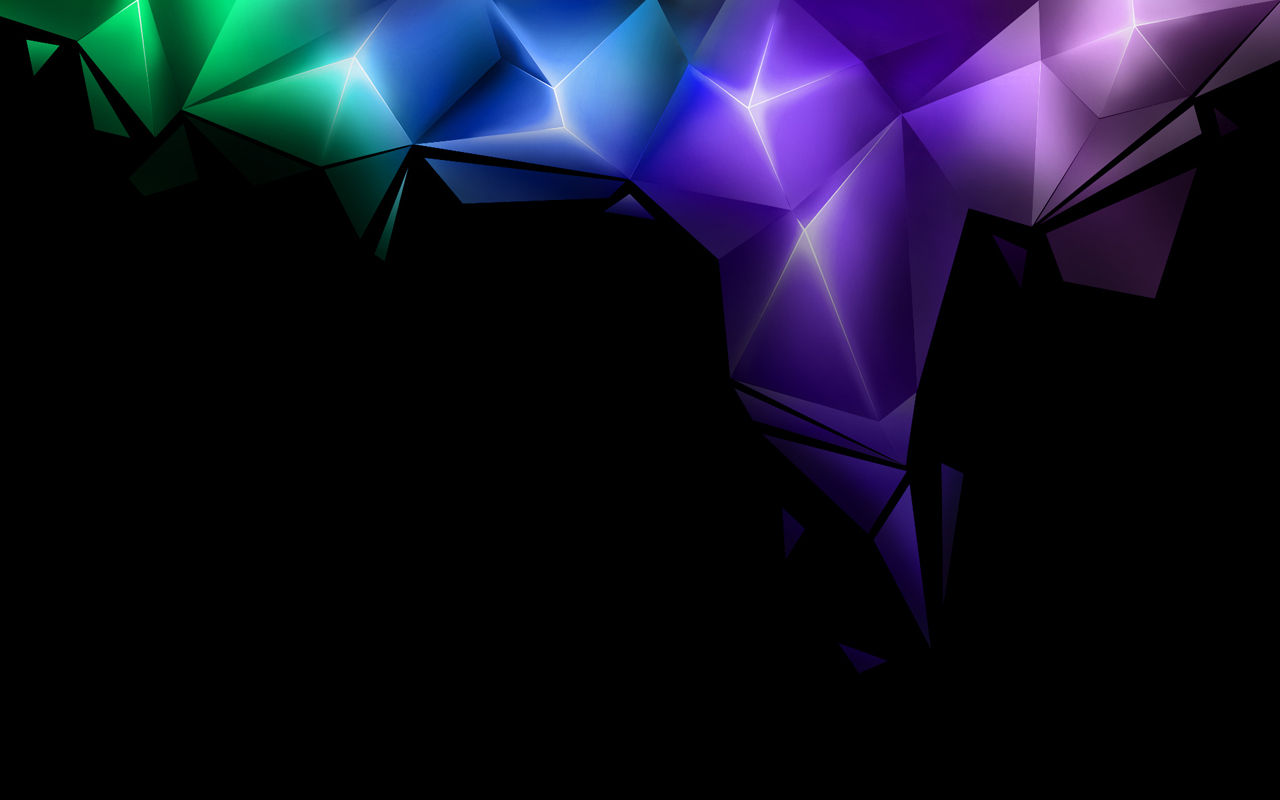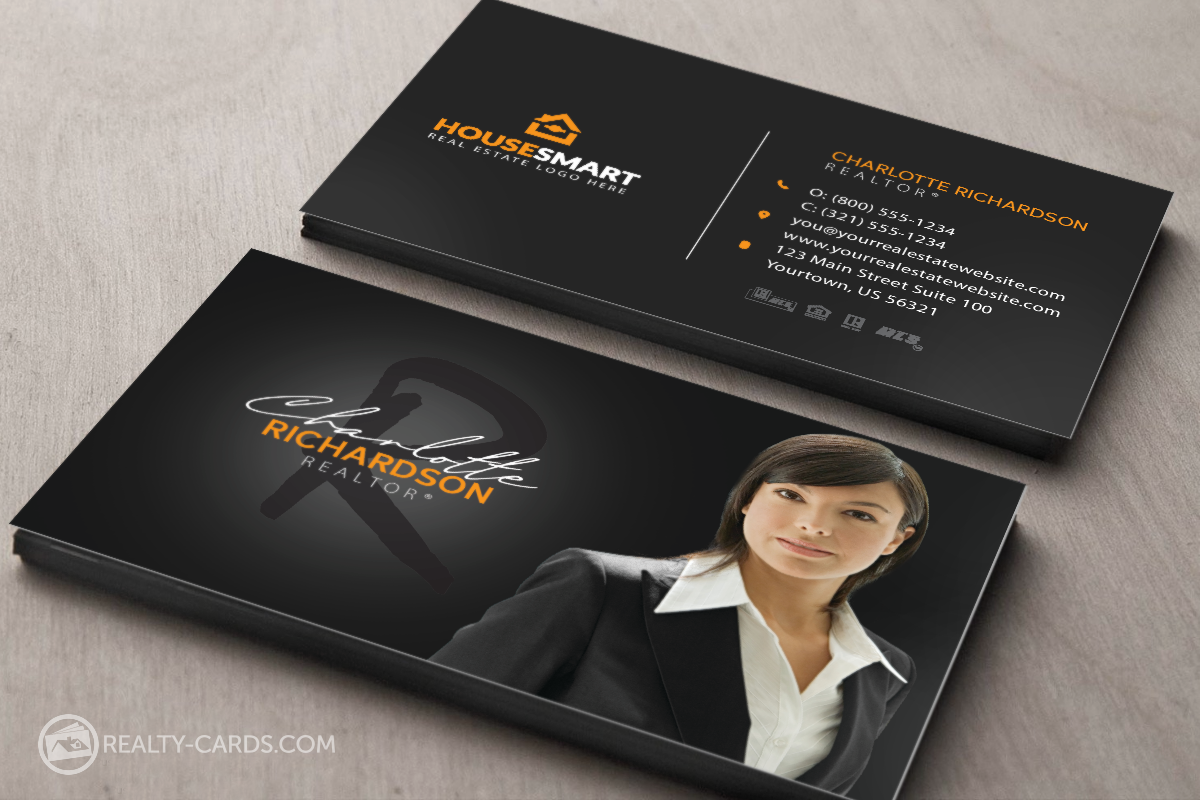top of pageQuantity | Price

500 =  \$180.00

1000 = \$290.00

2500 = \$460.00

5000 = \$835.00

Quantity | Price

500 = \$125.00

1000 = \$185.00

2500 = \$290.00

5000 = \$465.00

Quantity | Price

500 = \$85.00

1000 = \$130.00

2500 = \$215.00

5000 = \$385.00

Quantity | Price

500 = \$175.00

1000 = \$260.00

2500 = \$550.00

5000 = \$920.00

Quantity | Price

500 = \$140.00

1000 = \$260.00

2500 = \$380.00

5000 = \$640.00

Quantity | Price

500 = \$115.00

1000 = \$185.00

2500 = \$420.00

5000 = \$750.00

Quantity | Price

500 = \$50.00

1000 = \$65.00

2500 = \$95.00

5000 = \$170.00

Quantity | Price

500 = \$40.00

1000 = \$55.00

2500 = \$80.00

5000 = \$145.00

Quantity | Price

500 = \$65.00

1000 = \$105.00

2500 = \$250.00

5000 = \$450.00

## Invoices | 2 part NCRSize: 5.5 x 8.5 | Prints: BLACK, 1 over 0, 1 side 2 part NCR

Quantity | Price

500 = \$65.00

1000 = \$80.00

2500 = \$120.00

5000 = \$215.00Quantity | Price

500 = \$55.00

1000 = \$70.00

2500 = \$105.00

5000 = \$185.00## PostersSize: 12 x 18 | Prints: Color, 4 over 4, 1 side 10pt. White Cover C2S + UV Coating, 2 sides

Quantity | Price

1-25 = \$ 3.00 each

25-50 = \$ 2.00 each

Prices are for customers artwork saved as PDF, TIFF, JPEG or EPS files.

Quantity | Price

1000 = \$115.00

2000 = \$180.00

## Invoices | 2 part NCRSize: 8.5 x 11 | Prints: BLACK, 1 over 0, 1 side 2 part NCR

Quantity | Price

1000 = \$200.00

2000 = \$330.00Prices are for customers artwork saved as

PDF, TIFF, JPEG or EPS files.

Creating Artworks, Folding, Scoring, Numbering and shipping are to be charged extra for a small increase in price.

bottom of page